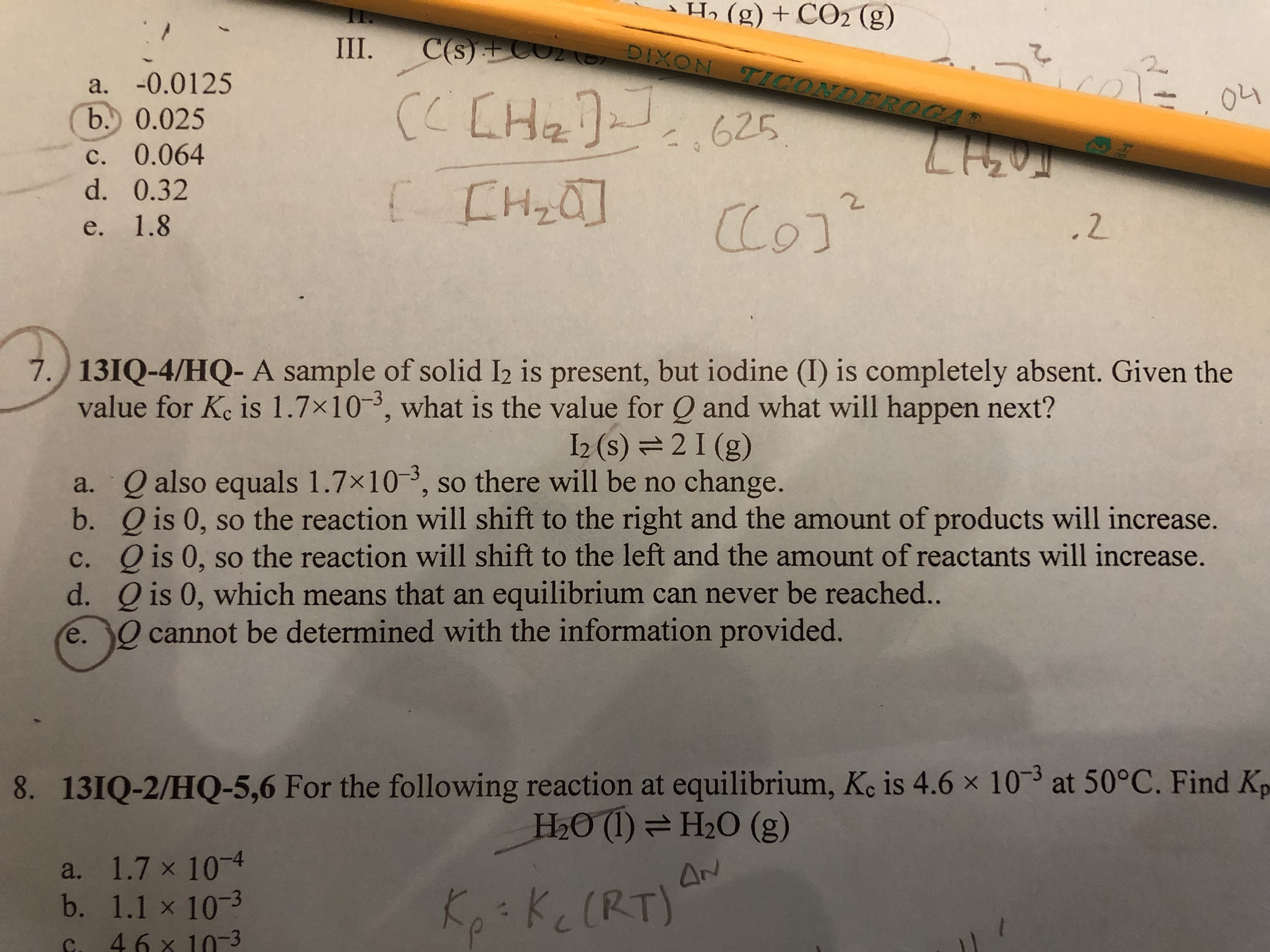# A sample of solid I2 is present, but iodine (I) is completely absent. Given the value for Kc is 1.7x10^-3, what is the value for Q and what will happen next? I2(s) <-> 2I (g) a. Q also equals 1.7x10^-3, so there will be no change. b. Q is 0, so the reaction will shift to the right and the amount of products will increase. c. Q is 0, so the reaction will shift to the left and the amount of the reactants will increase d. Q is 0, which means that an equilibrium can never be reached e. Q cannot be determined with the information provided